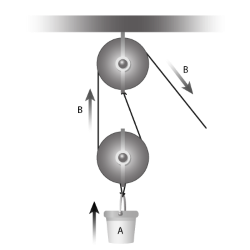• Browse All Lessons

Assign Lesson

Help Teaching subscribers can assign lessons to their students to review online!

 Tweet

PulleysIntroduction: To lift heavy loads, we often make use of pulleys, or simple machines comprised of a rope sliding around a disk called a block. Pulleys are especially important because they can change the direction or magnitude of an output force. Pulleys are particularly useful in that they reduce friction on a rope when lifting a load. In all pulleys, the input work always equals the output work. This is shown by the following equation:

$F_"in"D_"in"=F_"out"D_"out"$

where, $F_"in"=$"input force", $F_"out"=$"output force", $D_"in"=$"input distance", and $D_"out"=$"output distance"

Different types of pulleys are differentiated on the basis of their ideal mechanical advantage (IMA), which refers to the number of times that the force is multiplied under ideal conditions.

A single fixed pulley, for example, only changes the direction of the output force, having an IMA value of 1. In single movable pulleys, on the other hand, the output force is doubled, leading to an IMA value of 2. Finally, in pulley systems where there are 3 or more support ropes, the IMA value can range from 3 to higher values. In these pulley systems, the IMA value depends on the number of sections of rope pulling up on the object itself.

Required Video

Related Worksheets:

Related Lessons: